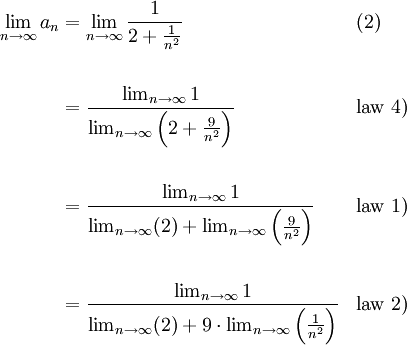# Limit Laws Example

Previous: Limit Laws for Infinite Sequences

Next: Relationship to Sequences of Absolute Values

## Example

Determine whether the sequenceconverges.

## Complete Solution

Using the limit laws for infinite sequence, we would evaluateBecause our limit evaluates to a finite number, the sequence converges (and it converges to 1/2).

The above example is trivial, but demonstrates why we need the limit laws. They allow us to evaluate limits of more complicated sequences.

## Explanation of Each Step

### Step (1)

We applied the definition of convergence of a sequence. Recall that to determine if a sequence is convergent we evaluateand if this limit exists, the sequence converges. If it doesn't the sequence is divergent.

### Step (2)

To make the limit easier to evaluate, we divided both the numerator and denominator by. This is as commonly used trick when evaluating limits.

### Step (3)### Steps (4, 5)

These two steps consisted of evaluating our limits and simple algebraic manipulation and should be straightforward to students who are familiar with limits of functions.

## Possible Challenges

### Memorizing the Limit Laws

Students are expected to memorize the laws, but not the labels we assigned to them. For example, students are expected to have memorized thatbut do not need to memorize that this is the third law.

Previous: Limit Laws for Infinite Sequences

Next: Relationship to Sequences of Absolute Values